# class TFormula: public TNamed

``` The FORMULA class

```
/*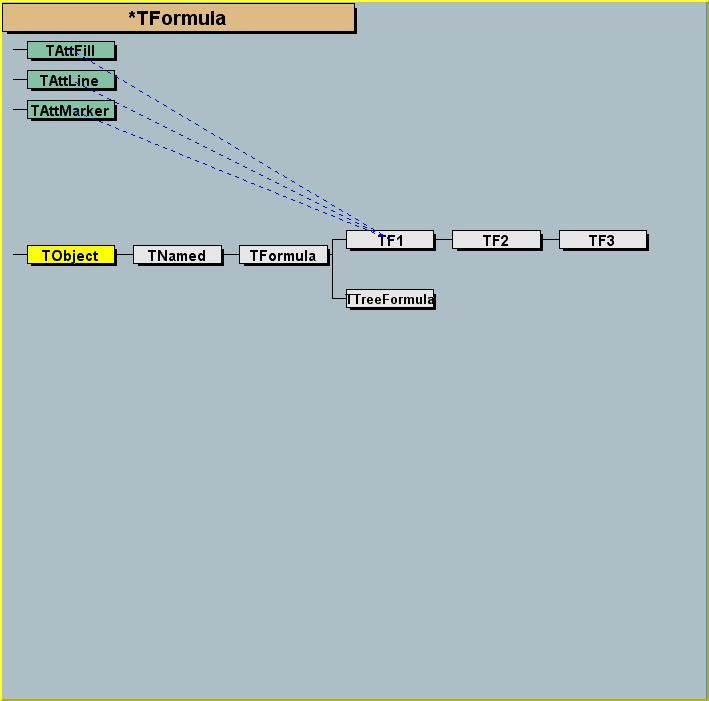*/
```
Example of valid expressions:
-  sin(x)/x
-  *sin(x) + *exp(-*x)
-  x + y**2
-  x^2 + y^2
-  *pow(,4)
-  2*pi*sqrt(x/y)
-  gaus(0)*expo(3)  + ypol3(5)*x
-  gausn(0)*expo(3) + ypol3(5)*x

In the last example above:
gaus(0) is a substitute for *exp(-0.5*((x-)/)**2)
and (0) means start numbering parameters at 0
gausn(0) is a substitute for *exp(-0.5*((x-)/)**2)/(sqrt(2*pi)*))
and (0) means start numbering parameters at 0
expo(3) is a substitute for exp(+*x)
pol3(5) is a substitute for par+par*x+par*x**2+par*x**3
(here Pol3 stands for Polynomial of degree 3)

TMath functions can be part of the expression, eg:
-  TMath::Landau(x)*sin(x)
-  TMath::Erf(x)

Comparisons operators are also supported (&&, ||, ==, <=, >=, !)
Examples:
sin(x*(x<0.5 || x>1))
If the result of a comparison is TRUE, the result is 1, otherwise 0.

Already predefined names can be given. For example, if the formula
TFormula old(sin(x*(x<0.5 || x>1))) one can assign a name to the formula. By default
the name of the object = title = formula itself.
old.SetName("old").
then, old can be reused in a new expression.
TFormula new("x*old") is equivalent to:
TFormula new("x*sin(x*(x<0.5 || x>1))")

Up to 4 dimensions are supported (indicated by x, y, z, t)
An expression may have 0 parameters or a list of parameters
indicated by the sequence [par_number]

A graph showing the logic to compile and analyze a formula
is shown in TFormula::Compile and TFormula::Analyze.
Once a formula has been compiled, it can be evaluated for a given
set of parameters. see graph in TFormula::EvalPar.

This class is the base class for the function classes TF1,TF2 and TF3.
It is also used by the ntuple selection mechanism TNtupleFormula.

In version 7 of TFormula, the usage of fOper has been changed
to improve the performance of TFormula::EvalPar.
Conceptually, fOper was changed from a simple array of Int_t
to an array of composite values.
For example a 'ylandau(5)' operation used to be encoded as 4105;
it is now encoded as (klandau >> kTFOperShit) + 5
Any class inheriting from TFormula and using directly fOper (which
is now a private data member), needs to be updated to take this
in consideration.  The member functions recommended to set and
access fOper are:  SetAction, GetAction, GetActionParam
TFormula::EvalPar

CHANGING DEFAULT SETTINGS

When creating complex formula , it may be necessary to increase
some default parameters. see static function TFormula::SetMaxima

WHY TFormula CANNOT ACCEPT A CLASS MEMBER FUNCTION ?

This is a frequently asked question.
C++ is a strongly typed language. There is no way for TFormula (without
recompiling this class) to know about all possible user defined data types.
This also apply to the case of a static class function.
Because TMath is a special and frequent case, TFormula is aware
of all TMath functions.
```

## Function Members (Methods)

public:
 virtual ~TFormula() void TObject::AbstractMethod(const char* method) const virtual void Analyze(const char* schain, Int_t& err, Int_t offset = 0) virtual Bool_t AnalyzeFunction(TString& chaine, Int_t& err, Int_t offset = 0) virtual void TObject::AppendPad(Option_t* option = "") virtual void TObject::Browse(TBrowser* b) static TClass* Class() virtual const char* TObject::ClassName() const virtual void Clear(Option_t* option = "") virtual TObject* TNamed::Clone(const char* newname = "") const virtual Int_t TNamed::Compare(const TObject* obj) const virtual Int_t Compile(const char* expression = "") virtual void Copy(TObject& formula) const virtual char* DefinedString(Int_t code) virtual Double_t DefinedValue(Int_t code) virtual Int_t DefinedVariable(TString& variable, Int_t& action) virtual void TObject::Delete(Option_t* option = "")MENU virtual Int_t TObject::DistancetoPrimitive(Int_t px, Int_t py) virtual void TObject::Draw(Option_t* option = "") virtual void TObject::DrawClass() constMENU virtual TObject* TObject::DrawClone(Option_t* option = "") constMENU virtual void TObject::Dump() constMENU virtual void TObject::Error(const char* method, const char* msgfmt) const virtual Double_t Eval(Double_t x, Double_t y = 0, Double_t z = 0, Double_t t = 0) const virtual Double_t EvalPar(const Double_t* x, const Double_t* params = 0) virtual Double_t EvalParOld(const Double_t* x, const Double_t* params = 0) virtual void TObject::Execute(const char* method, const char* params, Int_t* error = 0) virtual void TObject::Execute(TMethod* method, TObjArray* params, Int_t* error = 0) virtual void TObject::ExecuteEvent(Int_t event, Int_t px, Int_t py) virtual void TObject::Fatal(const char* method, const char* msgfmt) const virtual void TNamed::FillBuffer(char*& buffer) virtual TObject* TObject::FindObject(const char* name) const virtual TObject* TObject::FindObject(const TObject* obj) const virtual Option_t* TObject::GetDrawOption() const static Long_t TObject::GetDtorOnly() virtual TString GetExpFormula(Option_t* option = "") const virtual const char* TObject::GetIconName() const virtual const TObject* GetLinearPart(Int_t i) static void GetMaxima(Int_t& maxop, Int_t& maxpar, Int_t& maxconst) virtual const char* TNamed::GetName() const virtual Int_t GetNdim() const virtual Int_t GetNpar() const virtual Int_t GetNumber() const virtual char* TObject::GetObjectInfo(Int_t px, Int_t py) const static Bool_t TObject::GetObjectStat() virtual Option_t* TObject::GetOption() const Double_t GetParameter(Int_t ipar) const Double_t GetParameter(const char* name) const virtual Double_t* GetParameters() const virtual void GetParameters(Double_t* params) virtual const char* GetParName(Int_t ipar) const virtual Int_t GetParNumber(const char* name) const virtual const char* TNamed::GetTitle() const virtual UInt_t TObject::GetUniqueID() const virtual Bool_t TObject::HandleTimer(TTimer* timer) virtual ULong_t TNamed::Hash() const virtual void TObject::Info(const char* method, const char* msgfmt) const virtual Bool_t TObject::InheritsFrom(const char* classname) const virtual Bool_t TObject::InheritsFrom(const TClass* cl) const virtual void TObject::Inspect() constMENU void TObject::InvertBit(UInt_t f) virtual TClass* IsA() const virtual Bool_t TObject::IsEqual(const TObject* obj) const virtual Bool_t TObject::IsFolder() const virtual Bool_t IsLinear() const virtual Bool_t IsNormalized() const Bool_t TObject::IsOnHeap() const virtual Bool_t TNamed::IsSortable() const Bool_t TObject::IsZombie() const virtual void TNamed::ls(Option_t* option = "") const void TObject::MayNotUse(const char* method) const virtual Bool_t TObject::Notify() void TObject::Obsolete(const char* method, const char* asOfVers, const char* removedFromVers) const void TObject::operator delete(void* ptr) void TObject::operator delete(void* ptr, void* vp) void TObject::operator delete[](void* ptr) void TObject::operator delete[](void* ptr, void* vp) void* TObject::operator new(size_t sz) void* TObject::operator new(size_t sz, void* vp) void* TObject::operator new[](size_t sz) void* TObject::operator new[](size_t sz, void* vp) TFormula& operator=(const TFormula& rhs) void Optimize() virtual void TObject::Paint(Option_t* option = "") virtual void TObject::Pop() virtual void Print(Option_t* option = "") constMENU virtual void ProcessLinear(TString& replaceformula) virtual Int_t TObject::Read(const char* name) virtual void TObject::RecursiveRemove(TObject* obj) void TObject::ResetBit(UInt_t f) virtual void TObject::SaveAs(const char* filename = "", Option_t* option = "") constMENU virtual void TObject::SavePrimitive(ostream& out, Option_t* option = "") void TObject::SetBit(UInt_t f) void TObject::SetBit(UInt_t f, Bool_t set) virtual void TObject::SetDrawOption(Option_t* option = "")MENU static void TObject::SetDtorOnly(void* obj) static void SetMaxima(Int_t maxop = 1000, Int_t maxpar = 1000, Int_t maxconst = 1000) virtual void SetName(const char* name) virtual void TNamed::SetNameTitle(const char* name, const char* title) virtual void SetNumber(Int_t number) static void TObject::SetObjectStat(Bool_t stat) virtual void SetParameter(const char* name, Double_t parvalue) virtual void SetParameter(Int_t ipar, Double_t parvalue) virtual void SetParameters(const Double_t* params) virtual void SetParameters(Double_t p0, Double_t p1, Double_t p2 = 0, Double_t p3 = 0, Double_t p4 = 0, Double_t p5 = 0, Double_t p6 = 0, Double_t p7 = 0, Double_t p8 = 0, Double_t p9 = 0, Double_t p10 = 0)MENU virtual void SetParName(Int_t ipar, const char* name) virtual void SetParNames(const char* name0 = "p0", const char* name1 = "p1", const char* name2 = "p2", const char* name3 = "p3", const char* name4 = "p4", const char* name5 = "p5", const char* name6 = "p6", const char* name7 = "p7", const char* name8 = "p8", const char* name9 = "p9", const char* name10 = "p10")MENU virtual void TNamed::SetTitle(const char* title = "")MENU virtual void TObject::SetUniqueID(UInt_t uid) virtual void ShowMembers(TMemberInspector& insp) const virtual Int_t TNamed::Sizeof() const virtual void Streamer(TBuffer&) void StreamerNVirtual(TBuffer& ClassDef_StreamerNVirtual_b) virtual void TObject::SysError(const char* method, const char* msgfmt) const Bool_t TObject::TestBit(UInt_t f) const Int_t TObject::TestBits(UInt_t f) const TFormula() TFormula(const TFormula& formula) TFormula(const char* name, const char* formula) virtual void Update() virtual void TObject::UseCurrentStyle() virtual void TObject::Warning(const char* method, const char* msgfmt) const virtual Int_t TObject::Write(const char* name = 0, Int_t option = 0, Int_t bufsize = 0) virtual Int_t TObject::Write(const char* name = 0, Int_t option = 0, Int_t bufsize = 0) const
protected:
 virtual Bool_t CheckOperands(Int_t operation, Int_t& err) virtual Bool_t CheckOperands(Int_t leftoperand, Int_t rightoperartion, Int_t& err) void ClearFormula(Option_t* option = "") virtual void Convert(UInt_t fromVersion) virtual void TObject::DoError(int level, const char* location, const char* fmt, va_list va) const Double_t EvalParFast(const Double_t* x, const Double_t* params) Double_t EvalPrimitive(const Double_t* x, const Double_t* params) Double_t EvalPrimitive0(const Double_t* x, const Double_t* params) Double_t EvalPrimitive1(const Double_t* x, const Double_t* params) Double_t EvalPrimitive2(const Double_t* x, const Double_t* params) Double_t EvalPrimitive3(const Double_t* x, const Double_t* params) Double_t EvalPrimitive4(const Double_t* x, const Double_t* params) Short_t GetAction(Int_t code) const Short_t GetActionOptimized(Int_t code) const Int_t GetActionParam(Int_t code) const Int_t GetActionParamOptimized(Int_t code) const Int_t* GetOper() const Int_t* GetOperOptimized() const virtual Bool_t IsString(Int_t oper) const void MakePrimitive(const char* expr, Int_t pos) void TObject::MakeZombie() Int_t PreCompile() void SetAction(Int_t code, Int_t value, Int_t param = 0) void SetActionOptimized(Int_t code, Int_t value, Int_t param = 0) virtual Bool_t StringToNumber(Int_t code)

## Data Members

public:
 static TObject::(anonymous) TObject::kBitMask static TObject::EStatusBits TObject::kCanDelete static TObject::EStatusBits TObject::kCannotPick static TObject::EStatusBits TObject::kHasUUID static TObject::EStatusBits TObject::kInvalidObject static TObject::(anonymous) TObject::kIsOnHeap static TObject::EStatusBits TObject::kIsReferenced static TFormula::(anonymous) kLinear static TObject::EStatusBits TObject::kMustCleanup static TObject::EStatusBits TObject::kNoContextMenu static TFormula::(anonymous) kNormalized static TObject::(anonymous) TObject::kNotDeleted static TFormula::(anonymous) kNotGlobal static TObject::EStatusBits TObject::kObjInCanvas static TObject::(anonymous) TObject::kOverwrite static TObject::(anonymous) TObject::kSingleKey static TObject::(anonymous) TObject::kWriteDelete static TObject::(anonymous) TObject::kZombie
protected:
 TBits fAlreadyFound ! cache for information Double_t* fConst [fNconst] Array of fNconst formula constants TString* fExpr [fNoper] List of expressions TString* fExprOptimized ![fNOperOptimized] List of expressions TObjArray fFunctions Array of function calls to make TObjArray fLinearParts Linear parts if the formula is linear (contains '|' or "++") Int_t fNOperOptimized !Number of operators after optimization TString TNamed::fName object identifier TString* fNames [fNpar] Array of parameter names Int_t fNconst Number of constants Int_t fNdim Dimension of function (1=1-Dim, 2=2-Dim,etc) Int_t fNoper Number of operators Int_t fNpar Number of parameters Int_t fNstring Number of different constants character strings Int_t fNumber formula number identifier Int_t fNval Number of different variables in expression TOperOffset* fOperOffset ![fNOperOptimized] Offsets of operrands Int_t* fOperOptimized ![fNOperOptimized] List of operators. (See documentation for changes made at version 7) TFormula::TFuncG fOptimal !pointer to optimal function Double_t* fParams [fNpar] Array of fNpar parameters TFormulaPrimitive** fPredefined ![fNPar] predefined function TString TNamed::fTitle object title static TFormula::(anonymous) kAdd static TFormula::(anonymous) kAnd static TFormula::(anonymous) kBinary static TFormula::(anonymous) kBitAnd static TFormula::(anonymous) kBitOr static TFormula::(anonymous) kBoolOptimize static TFormula::(anonymous) kBoolOptimizeAnd static TFormula::(anonymous) kBoolOptimizeOr static TFormula::(anonymous) kBoolSet static TFormula::(anonymous) kConstant static TFormula::(anonymous) kData static TFormula::(anonymous) kDefinedString static TFormula::(anonymous) kDefinedVariable static TFormula::(anonymous) kDivide static TFormula::(anonymous) kEnd static TFormula::(anonymous) kEqual static TFormula::(anonymous) kFD0 static TFormula::(anonymous) kFD1 static TFormula::(anonymous) kFD2 static TFormula::(anonymous) kFD3 static TFormula::(anonymous) kFDM static TFormula::(anonymous) kFunctionCall static TFormula::(anonymous) kGreater static TFormula::(anonymous) kGreaterThan static TFormula::(anonymous) kJump static TFormula::(anonymous) kJumpIf static TFormula::(anonymous) kLeftShift static TFormula::(anonymous) kLess static TFormula::(anonymous) kLessThan static TFormula::(anonymous) kModulo static TFormula::(anonymous) kMultD static TFormula::(anonymous) kMultDD static TFormula::(anonymous) kMultiply static TFormula::(anonymous) kNot static TFormula::(anonymous) kNotEqual static TFormula::(anonymous) kOr static TFormula::(anonymous) kParameter static TFormula::(anonymous) kPlusD static TFormula::(anonymous) kPlusDD static TFormula::(anonymous) kRightShift static TFormula::(anonymous) kSignInv static TFormula::(anonymous) kStringConst static TFormula::(anonymous) kStringEqual static TFormula::(anonymous) kStringNotEqual static TFormula::(anonymous) kSubstract static TFormula::(anonymous) kThree static TFormula::(anonymous) kUnary static TFormula::(anonymous) kVariable static TFormula::(anonymous) kabs static TFormula::(anonymous) kacos static TFormula::(anonymous) kacosh static TFormula::(anonymous) kasin static TFormula::(anonymous) kasinh static TFormula::(anonymous) katan static TFormula::(anonymous) katan2 static TFormula::(anonymous) katanh static TFormula::(anonymous) kcos static TFormula::(anonymous) kcosh static TFormula::(anonymous) kexp static TFormula::(anonymous) kexpo static TFormula::(anonymous) kfmod static TFormula::(anonymous) kgaus static TFormula::(anonymous) kint static TFormula::(anonymous) klandau static TFormula::(anonymous) klog static TFormula::(anonymous) klog10 static TFormula::(anonymous) kmax static TFormula::(anonymous) kmin static TFormula::(anonymous) kpi static TFormula::(anonymous) kpol static TFormula::(anonymous) kpow static TFormula::(anonymous) krndm static TFormula::(anonymous) ksign static TFormula::(anonymous) ksin static TFormula::(anonymous) ksinh static TFormula::(anonymous) ksq static TFormula::(anonymous) ksqrt static TFormula::(anonymous) kstrstr static TFormula::(anonymous) ktan static TFormula::(anonymous) ktanh static TFormula::(anonymous) kxexpo static TFormula::(anonymous) kxgaus static TFormula::(anonymous) kxlandau static TFormula::(anonymous) kxpol static TFormula::(anonymous) kxyexpo static TFormula::(anonymous) kxygaus static TFormula::(anonymous) kxylandau static TFormula::(anonymous) kyexpo static TFormula::(anonymous) kygaus static TFormula::(anonymous) kylandau static TFormula::(anonymous) kypol static TFormula::(anonymous) kzexpo static TFormula::(anonymous) kzgaus static TFormula::(anonymous) kzlandau static TFormula::(anonymous) kzpol
private:
 Int_t* fOper [fNoper] List of operators. (See documentation for changes made at version 7)

## Class Charts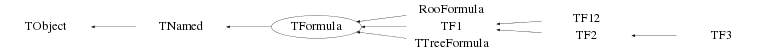## Function documentation

TFormula()
``` Formula default constructor.
```
TFormula(const char* name, const char* formula)
``` Normal Formula constructor.
```
TFormula(const TFormula& formula)
``` Default constructor.
```
TFormula& operator=(const TFormula& rhs)
``` Operator =
```

``` Formula default destructor.
```
Bool_t AnalyzeFunction(TString& chaine, Int_t& err, Int_t offset = 0)
``` Check if the chain as function call.

If you overload this member function, you also HAVE TO
never call the constructor:

TFormula::TFormula(const char *name,const char *expression)

MyClass::MyClass(const char *name,const char *expression) : TFormula()

which has to call the TFormula default constructor and whose implementation
should be similar to the implementation of the normal TFormula constructor

This is necessary because the normal TFormula constructor call indirectly
the virtual member functions Analyze, DefaultString, DefaultValue
and DefaultVariable.
```
void Analyze(const char* schain, Int_t& err, Int_t offset = 0)
``` Analyze a sub-expression in one formula.

Expressions in one formula are recursively analyzed.
Result of analysis is stored in the object tables.

Table of function codes and errors

* functions :

+           1                   pow          20
-           2                   sq           21
*           3                   sqrt         22
/           4                   strstr       23
%           5                   min          24
max          25
log          30
cos         10                  exp          31
sin         11                  log10        32
tan         12
acos        13                  abs          41
asin        14                  sign         42
atan        15                  int          43
atan2       16
fmod        17                  rndm         50

cosh        70                  acosh        73
sinh        71                  asinh        74
tanh        72                  atanh        75

expo       100                  gaus        110     gausn  (see note below)
expo(0)    100 0                gaus(0)     110 0   gausn(0)
expo(1)    100 1                gaus(1)     110 1   gausn(1)
xexpo      100 x                xgaus       110 x   xgausn
yexpo      101 x                ygaus       111 x   ygausn
zexpo      102 x                zgaus       112 x   zgausn
xyexpo     105 x                xygaus      115 x   xygausn
yexpo(5)   102 5                ygaus(5)    111 5   ygausn(5)
xyexpo(2)  105 2                xygaus(2)   115 2   xygausn(2)

landau      120 x   landaun (see note below)
landau(0)   120 0   landaun(0)
landau(1)   120 1   landaun(1)
xlandau     120 x   xlandaun
ylandau     121 x   ylandaun
zlandau     122 x   zlandaun
xylandau    125 x   xylandaun
ylandau(5)  121 5   ylandaun(5)
xylandau(2) 125 2   xylandaun(2)

pol0        130 x               pol1        130 1xx
pol0(0)     130 0               pol1(0)     130 100
pol0(1)     130 1               pol1(1)     130 101
xpol0       130 x               xpol1       130 101
ypol0       131 x               ypol1       131 101
zpol0       132 x               zpol1       132 1xx
ypol0(5)    131 5               ypol1(5)    131 105

pi          40

&&          60                  <            64
||          61                  >            65
==          62                  <=           66
!=          63                  =>           67
!           68
==(string)  76                  &            78
!=(string)  77                  |            79
<<(shift)   80                  >>(shift)    81
? :         82

* constants (kConstants) :

c0  141 1      c1  141 2  etc..

* strings (kStringConst):

sX  143 x

* variables (kFormulaVar) :

x    144 0      y    144 1      z    144 2      t    144 3

* parameters :

        140 1
        140 2
etc.

Special cases for normalized gaussian or landau distributions

the expression "gaus" is a substitute for
*exp(-0.5*((x-)/)**2)
to obtain a standard normalized gaussian, use "gausn" instead of "gaus"
the expression "gausn" is a substitute for
*exp(-0.5*((x-)/)**2)/(sqrt(2*pi)*))
WARNING: gaus and gausn are mutually exclusive in the same expression.

In the same way the expression "landau" is a substitute for
*TMath::Landau(x,,,kFALSE)
to obtain a standard normalized landau, use "landaun" instead of "landau"
the expression "landaun" is a substitute for
*TMath::Landau(x,,,kTRUE)
WARNING: landau and landaun are mutually exclusive in the same expression.

Boolean optimization (kBoolOptmize) :

Those pseudo operation are used to implement lazy evaluation of
&& and ||.  When the left hand of the expression if false
(respectively true), the evaluation of the right is entirely skipped
(since it would not change the value of the expreession).

&&   142 11 (one operation on right) 142 21 (2 operations on right)
||   142 12 (one operation on right) 142 22 (2 operations on right)

* functions calls (kFunctionCall) :

f0 145  0  f1 145  1  etc..

Errors :

1  : Division By Zero
2  : Invalid Floating Point Operation
4  : Empty String
5  : invalid syntax
6  : Too many operators
7  : Too many parameters
10  : z specified but not x and y
11  : z and y specified but not x
12  : y specified but not x
13  : z and x specified but not y
20  : non integer value for parameter number
21  : atan2 requires two arguments
22  : pow requires two arguments
23  : degree of polynomial not specified
24  : Degree of polynomial must be positive
25  : Degree of polynomial must be less than 20
26  : Unknown name
27  : Too many constants in expression
28  : strstr requires two arguments
29  : interpreted or compiled function have to return a numerical type
31  : Part of the variable exist but some of it is not accessible or useable
40  : '(' is expected
41  : ')' is expected
42  : '[' is expected
43  : ']' is expected

```
/*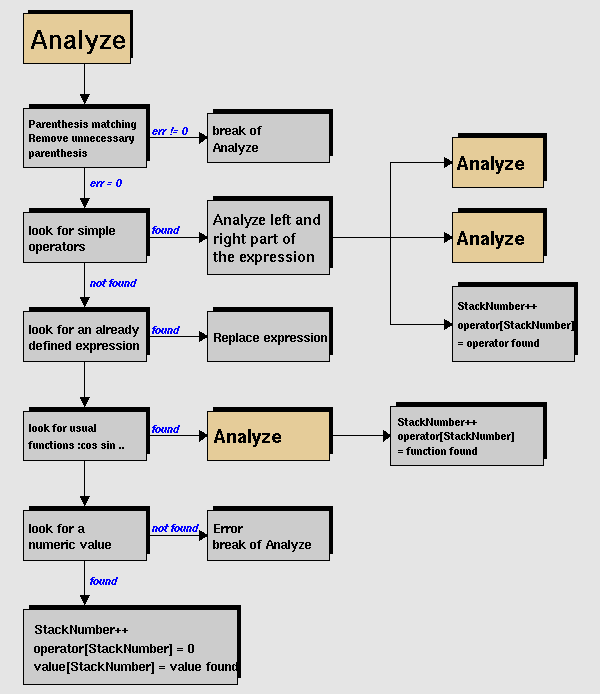*/
```
Special functions

By default, the formula is assigned fNumber=0. However, the following
formula built with simple functions are assigned  fNumber:
"gaus"      100  (or gausn)
"xygaus"    110
"expo"      200
"polN"      300+N
"landau"    400
"xylandau"  410
Note that expressions like gaus(0), expo(1) will force fNumber=0

Warning when deriving a class from TFormula

If you overload this member function, you also HAVE TO
never call the constructor:

TFormula::TFormula(const char *name,const char *expression)

MyClass::MyClass(const char *name,const char *expression) : TFormula()

which has to call the TFormula default constructor and whose implementation
should be similar to the implementation of the normal TFormula constructor

This is necessary because the normal TFormula constructor call indirectly
the virtual member functions Analyze, DefaultString, DefaultValue
and DefaultVariable.
```
Bool_t CheckOperands(Int_t operation, Int_t& err)
``` Check whether the operand at 'oper-1' is compatible with the operation
at 'oper'.
```
Bool_t CheckOperands(Int_t leftoperand, Int_t rightoperartion, Int_t& err)
``` Check whether the operands at 'leftoper' and 'oper-1' are compatible with
the operation at 'oper'.
```

``` Try to 'demote' a string into an array bytes.  If this is not possible,
return false.
```
void Clear(Option_t* option = "")
``` Resets the objects.

Resets the object to its state before compilation.
```
void ClearFormula(Option_t* option = "")
``` Resets the objects.

Resets the object to its state before compilation.
```
Int_t Compile(const char* expression = "")
``` Compile expression already stored in fTitle.

Loop on all subexpressions of formula stored in fTitle

If you overload this member function, you also HAVE TO
never call the constructor:

TFormula::TFormula(const char *name,const char *expression)

MyClass::MyClass(const char *name,const char *expression) : TFormula()

which has to call the TFormula default constructor and whose implementation
should be similar to the implementation of the normal TFormula constructor

This is necessary because the normal TFormula constructor call indirectly
the virtual member functions Analyze, DefaultString, DefaultValue
and DefaultVariable.

```
/*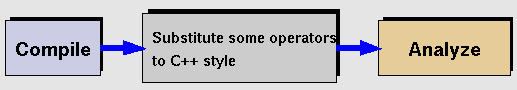*/
void Copy(TObject& formula) const
``` Copy this formula.
```
char * DefinedString(Int_t code)
``` Return address of string corresponding to special code.

This member function is inactive in the TFormula class.
It may be redefined in derived classes.

If you overload this member function, you also HAVE TO
never call the constructor:

TFormula::TFormula(const char *name,const char *expression)

MyClass::MyClass(const char *name,const char *expression) : TFormula()

which has to call the TFormula default constructor and whose implementation
should be similar to the implementation of the normal TFormula constructor

This is necessary because the normal TFormula constructor call indirectly
the virtual member functions Analyze, DefaultString, DefaultValue
and DefaultVariable.
```

``` Return value corresponding to special code.

This member function is inactive in the TFormula class.
It may be redefined in derived classes.

If you overload this member function, you also HAVE TO
never call the constructor:

TFormula::TFormula(const char *name,const char *expression)

MyClass::MyClass(const char *name,const char *expression) : TFormula()

which has to call the TFormula default constructor and whose implementation
should be similar to the implementation of the normal TFormula constructor

This is necessary because the normal TFormula constructor call indirectly
the virtual member functions Analyze, DefaultString, DefaultValue
and DefaultVariable.
```
Int_t DefinedVariable(TString& variable, Int_t& action)
``` Check if expression is in the list of defined variables.

This member function can be overloaded in derived classes

If you overload this member function, you also HAVE TO
never call the constructor:

TFormula::TFormula(const char *name,const char *expression)

MyClass::MyClass(const char *name,const char *expression) : TFormula()

which has to call the TFormula default constructor and whose implementation
should be similar to the implementation of the normal TFormula constructor

This is necessary because the normal TFormula constructor call indirectly
the virtual member functions Analyze, DefaultString, DefaultValue
and DefaultVariable.

The expected returns values are
-2 :  the name has been recognized but won't be usable
-1 :  the name has not been recognized
>=0 :  the name has been recognized, return the action parameter.
```
Double_t Eval(Double_t x, Double_t y = 0, Double_t z = 0, Double_t t = 0) const
``` Evaluate this formula.

The current value of variables x,y,z,t is passed through x, y, z and t.
The parameters used will be the ones in the array params if params is given
otherwise parameters will be taken from the stored data members fParams
```
Double_t EvalParOld(const Double_t* x, const Double_t* params = 0)
``` Evaluate this formula.

The current value of variables x,y,z,t is passed through the pointer x.
The parameters used will be the ones in the array params if params is given
otherwise parameters will be taken from the stored data members fParams

```
/*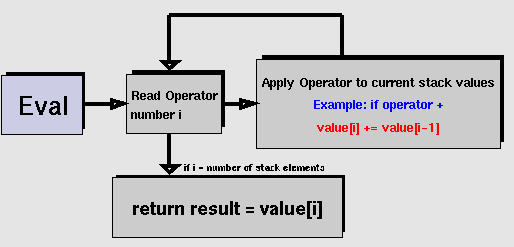*/
TString GetExpFormula(Option_t* option = "") const
``` Reconstruct the formula expression from the internal TFormula member variables

This function uses the internal member variables of TFormula to
construct the mathematical expression associated with the TFormula
instance. This function can be used to get an expanded version of the
expression originally assigned to the TFormula instance, i.e. that
the string returned by GetExpFormula() doesn't depend on other
TFormula object names.

if option contains "p" the returned string will contain the formula
expression with symbolic parameters, eg  replaced by the actual value
of the parameter. Example:
if expression in formula is: "*(x>-)+*exp(-*x)"
and parameters are 3.25,-4.01,4.44,-0.04, GetExpFormula("p") will return:
"(3.25*(x>+4.01))+(4.44*exp(+0.04*x))"
```
const TObject* GetLinearPart(Int_t i)
``` Return linear part.
```
Double_t GetParameter(Int_t ipar) const
``` Return value of parameter number ipar.
```
Double_t GetParameter(const char* name) const
``` Return value of parameter named parName.
```
const char * GetParName(Int_t ipar) const
``` Return name of one parameter.
```
Int_t GetParNumber(const char* name) const
``` Return parameter number by name.
```
Bool_t IsString(Int_t oper) const
``` Return true if the expression at the index 'oper' has to be treated as a string
```
void Print(Option_t* option = "") const
``` Dump this formula with its attributes.
```
void ProcessLinear(TString& replaceformula)
``` If the formula is for linear fitting, change the title to
normal and fill the LinearParts array
```
void SetName(const char* name)
``` Set the name of the formula. We need to allow the list of function to
properly handle the hashes.
```
void SetParameter(const char* name, Double_t parvalue)
``` Initialize parameter number ipar.
```
void SetParameter(Int_t ipar, Double_t parvalue)
``` Initialize parameter number ipar.
```
void SetParameters(const Double_t* params)
``` Initialize array of all parameters.
```
void SetParameters(Double_t p0, Double_t p1, Double_t p2 = 0, Double_t p3 = 0, Double_t p4 = 0, Double_t p5 = 0, Double_t p6 = 0, Double_t p7 = 0, Double_t p8 = 0, Double_t p9 = 0, Double_t p10 = 0)
``` Initialize up to 11 parameters
All arguments except THE FIRST TWO are optional
In case of a function with only one parameter, call this function with p1=0.
Minimum two arguments are required to differentiate this function
from the SetParameters(cont Double_t *params)
```
void SetParName(Int_t ipar, const char* name)
``` Set name of parameter number ipar
```
void SetParNames(const char* name0 = "p0", const char* name1 = "p1", const char* name2 = "p2", const char* name3 = "p3", const char* name4 = "p4", const char* name5 = "p5", const char* name6 = "p6", const char* name7 = "p7", const char* name8 = "p8", const char* name9 = "p9", const char* name10 = "p10")
``` Set up to 11 parameter names.
```
void Streamer(TBuffer& )
``` Stream a class object.
```
void Convert(UInt_t fromVersion)
``` Convert the fOper of a TFormula version fromVersion to the current in memory version
```
void MakePrimitive(const char* expr, Int_t pos)
```  MakePrimitive
find TFormulaPrimitive replacement for some operands
```
void Optimize()
``` MI include

Optimize formula
1.) Minimize the number of operands
a.)  several operanands are glued togther
b.)  some primitive functions glued together - exemp. (x+y) => PlusXY(x,y)
c.)  maximize number of standard calls minimizing number of jumps in Eval cases
d.)  variables, parameters and constants are mapped - using fOperOfssets0
Eval procedure use direct acces to data (only one corresponding case statement in eval procedure)

pdata[operand={Var,Par,Const}][offset]
pdata[fOperOffsets0[i]][fOperOffset1[i+1]]
2.) The fastest evaluation function is chosen at the end
a.) fOptimal := pointer to the fastest function for given evaluation string
switch(GetActionOptimized(0)){
case kData : {fOptimal= (TFormulaPrimitive::TFuncG)&TFormula::EvalPrimitive0; break;}
case kUnary : {fOptimal= (TFormulaPrimitive::TFuncG)&TFormula::EvalPrimitive1; break;}
case kBinary : {fOptimal= (TFormulaPrimitive::TFuncG)&TFormula::EvalPrimitive2; break;}
case kThree : {fOptimal= (TFormulaPrimitive::TFuncG)&TFormula::EvalPrimitive3; break;}
case kFDM : {fOptimal= (TFormulaPrimitive::TFuncG)&TFormula::EvalPrimitive4; break;}
}
b.) ex. fOptimal = ::EvalPrimitive0 - if it return only variable, constant or parameter
= ::EvalParameter1 - if only one unary operation
= ::EvalPrimitive2 - if only one binary operation

```
Double_t EvalPrimitive(const Double_t* x, const Double_t* params)
``` Evaluate primitive formula
```
Double_t EvalPrimitive0(const Double_t* x, const Double_t* params)
``` Evaluate primitive formula
```
Double_t EvalPrimitive1(const Double_t* x, const Double_t* params)
``` Evaluate primitive formula
```
Double_t EvalPrimitive2(const Double_t* x, const Double_t* params)
``` Evaluate primitive formula
```
Double_t EvalPrimitive3(const Double_t* x, const Double_t* params)
``` Evaluate primitive formula
```
Double_t EvalPrimitive4(const Double_t* x, const Double_t* params)
``` Evaluate primitive formula
```
Double_t EvalParFast(const Double_t* x, const Double_t* params)
``` Evaluate this formula.

The current value of variables x,y,z,t is passed through the pointer x.
The parameters used will be the ones in the array params if params is given
otherwise parameters will be taken from the stored data members fParams

```
/**/

``` Pre compile function
```
void SetMaxima(Int_t maxop = 1000, Int_t maxpar = 1000, Int_t maxconst = 1000)
``` static function to set the maximum value of 3 parameters
-maxop    : maximum number of operations
-maxpar   : maximum number of parameters
-maxconst : maximum number of constants
None of these parameters cannot be less than 10 (default is 1000)
call this function to increase one or all maxima when processing
very complex formula, eg TFormula::SetMaxima(100000,1000,1000000);
If you process many functions with a small number of operations/parameters
you may gain some memory and performance by decreasing these values.
```
void GetMaxima(Int_t& maxop, Int_t& maxpar, Int_t& maxconst)
``` static function to get the maximum value of 3 parameters
-maxop    : maximum number of operations
-maxpar   : maximum number of parameters
-maxconst : maximum number of constants
```
Int_t * GetOper() const
`{ return fOper; }`
Short_t GetAction(Int_t code) const
`{ return fOper[code] >> kTFOperShift; }`
Int_t GetActionParam(Int_t code) const
`{ return fOper[code] & kTFOperMask; }`
void SetAction(Int_t code, Int_t value, Int_t param = 0)
Int_t * GetOperOptimized() const
`{ return fOperOptimized; }`
Short_t GetActionOptimized(Int_t code) const
`{ return fOperOptimized[code] >> kTFOperShift; }`
Int_t GetActionParamOptimized(Int_t code) const
`{ return fOperOptimized[code] & kTFOperMask; }`
void SetActionOptimized(Int_t code, Int_t value, Int_t param = 0)
Double_t EvalPar(const Double_t* x, const Double_t* params = 0)
`{return ((*this).*fOptimal)(x,params);}`
Int_t GetNdim() const
`{return fNdim;}`
Int_t GetNpar() const
`{return fNpar;}`
Int_t GetNumber() const
`{return fNumber;}`
Double_t * GetParameters() const
`{return fParams;}`
void GetParameters(Double_t* params)
`{for(Int_t i=0;i<fNpar;i++) params[i] = fParams[i];}`
Bool_t IsLinear() const
`{return TestBit(kLinear);}`
Bool_t IsNormalized() const
`{return TestBit(kNormalized);}`
void SetNumber(Int_t number)
`{fNumber = number;}`
void Update()
`{;}`